Updating search results...

# 108 Results

View
Selected filters:
• Statistics and Probability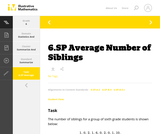Unrestricted Use
CC BY
Rating
0.0 stars

This is a task from the Illustrative Mathematics website that is one part of a complete illustration of the standard to which it is aligned. Each task has at least one solution and some commentary that addresses important aspects of the task and its potential use.

Subject:
Statistics and Probability
Material Type:
Activity/Lab
Provider:
Illustrative Mathematics
Provider Set:
Illustrative Mathematics
Author:
Illustrative Mathematics
08/06/2015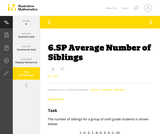Unrestricted Use
CC BY
Rating
0.0 stars

This is a task from the Illustrative Mathematics website that is one part of a complete illustration of the standard to which it is aligned. Each task has at least one solution and some commentary that addresses important aspects of the task and its potential use.

Subject:
Statistics and Probability
Material Type:
Activity/Lab
Provider:
Illustrative Mathematics
Provider Set:
Illustrative Mathematics
Author:
Illustrative Mathematics
08/06/2015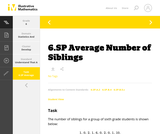Unrestricted Use
CC BY
Rating
0.0 stars

This is a task from the Illustrative Mathematics website that is one part of a complete illustration of the standard to which it is aligned. Each task has at least one solution and some commentary that addresses important aspects of the task and its potential use.

Subject:
Statistics and Probability
Material Type:
Activity/Lab
Provider:
Illustrative Mathematics
Provider Set:
Illustrative Mathematics
Author:
Illustrative Mathematics
08/06/2015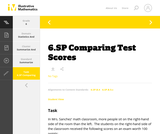Unrestricted Use
CC BY
Rating
0.0 stars

This is a task from the Illustrative Mathematics website that is one part of a complete illustration of the standard to which it is aligned. Each task has at least one solution and some commentary that addresses important aspects of the task and its potential use.

Subject:
Statistics and Probability
Material Type:
Activity/Lab
Provider:
Illustrative Mathematics
Provider Set:
Illustrative Mathematics
Author:
Illustrative Mathematics
08/06/2015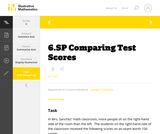Unrestricted Use
CC BY
Rating
0.0 stars

This is a task from the Illustrative Mathematics website that is one part of a complete illustration of the standard to which it is aligned. Each task has at least one solution and some commentary that addresses important aspects of the task and its potential use.

Subject:
Statistics and Probability
Material Type:
Activity/Lab
Provider:
Illustrative Mathematics
Provider Set:
Illustrative Mathematics
Author:
Illustrative Mathematics
08/06/2015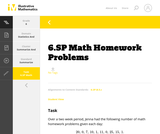Unrestricted Use
CC BY
Rating
0.0 stars

This is a task from the Illustrative Mathematics website that is one part of a complete illustration of the standard to which it is aligned. Each task has at least one solution and some commentary that addresses important aspects of the task and its potential use.

Subject:
Statistics and Probability
Material Type:
Activity/Lab
Provider:
Illustrative Mathematics
Provider Set:
Illustrative Mathematics
Author:
Illustrative Mathematics
08/06/2015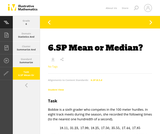Unrestricted Use
CC BY
Rating
0.0 stars

This is a task from the Illustrative Mathematics website that is one part of a complete illustration of the standard to which it is aligned. Each task has at least one solution and some commentary that addresses important aspects of the task and its potential use.

Subject:
Statistics and Probability
Material Type:
Activity/Lab
Provider:
Illustrative Mathematics
Provider Set:
Illustrative Mathematics
Author:
Illustrative Mathematics
08/06/2015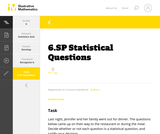Unrestricted Use
CC BY
Rating
0.0 stars

This is a task from the Illustrative Mathematics website that is one part of a complete illustration of the standard to which it is aligned. Each task has at least one solution and some commentary that addresses important aspects of the task and its potential use.

Subject:
Statistics and Probability
Material Type:
Activity/Lab
Provider:
Illustrative Mathematics
Provider Set:
Illustrative Mathematics
Author:
Illustrative Mathematics
08/06/2015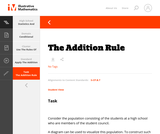Unrestricted Use
CC BY
Rating
0.0 stars

This is a task from the Illustrative Mathematics website that is one part of a complete illustration of the standard to which it is aligned. Each task has at least one solution and some commentary that addresses important aspects of the task and its potential use.

Subject:
Statistics and Probability
Material Type:
Activity/Lab
Provider:
Illustrative Mathematics
Provider Set:
Illustrative Mathematics
Author:
Illustrative Mathematics
08/06/2015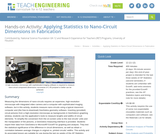Educational Use
Rating
0.0 stars

Measuring the dimensions of nano-circuits requires an expensive, high-resolution microscope with integrated video camera and a computer with sophisticated imaging software, but in this activity, students measure nano-circuits using a typical classroom computer and (the free-to-download) GeoGebra geometry software. Inserting (provided) circuit pictures from a high-resolution microscope as backgrounds in GeoGebra's graphing window, students use the application's tools to measure lengths and widths of circuit elements. To simplify the conversion from the on-screen units to the real circuits' units and the manipulation of the pictures, a GeoGebra measuring interface is provided. Students export their data from GeoGebra to Microsoft® Excel® for graphing and analysis. They test the statistical significance of the difference in circuit dimensions, as well as obtain a correlation between average changes in original vs. printed circuits' widths. This activity and its associated lesson are suitable for use during the last six weeks of the AP Statistics course; see the topics and timing note below for details.

Subject:
Mathematics
Statistics and Probability
Material Type:
Activity/Lab
Provider:
TeachEngineering
Provider Set:
TeachEngineering
Author:
Cunjiang Yu
Miguel R. Ramirez
Minwei Xu
Song Chen
02/17/2017Unrestricted Use
CC BY
Rating
2.0 stars

This resource consists of a Java applet and expository text. The applet is a simulation of the ballot experiment: The votes in an election are randomly counted. The event of interest is that the winning candidate is always ahead in the vote count.

Subject:
Statistics and Probability
Material Type:
Activity/Lab
Interactive
Simulation
Provider:
University of Alabama in Huntsville
Provider Set:
Virtual Laboratories in Probability and Statistics
Author:
Kyle Siegrist
02/16/2011Unrestricted Use
CC BY
Rating
0.0 stars

This resource consists of a Java applet and expository text. The applet is a simulation of Bertrand's experiment: a random chord on a circle The event of interest is whether the length of the chord is larger than the length of the inscribed equilateral triangle. Three models for generating the random chord can be used.

Subject:
Statistics and Probability
Material Type:
Activity/Lab
Interactive
Simulation
Provider:
University of Alabama in Huntsville
Provider Set:
Virtual Laboratories in Probability and Statistics
Author:
Kyle Siegrist
02/16/2011Unrestricted Use
CC BY
Rating
0.0 stars

This resource consists of a Java applet and expository text. The applet illustrates Bayesian estimation of the probability of heads for a coin. The prior beta distribution, true probability of heads, and the sample size can be specified. The applet shows the posterior beta distribution.

Subject:
Statistics and Probability
Material Type:
Activity/Lab
Interactive
Simulation
Provider:
University of Alabama in Huntsville
Provider Set:
Virtual Laboratories in Probability and Statistics
Author:
Kyle Siegrist
02/16/2011Unrestricted Use
CC BY
Rating
0.0 stars

This resource consists of a Java applet and expository text. The applet simulates a random sample from a beta distribution, and computes standard point estimates of the left and right parameters. The bias and mean square error are also computed.

Subject:
Statistics and Probability
Material Type:
Activity/Lab
Interactive
Simulation
Provider:
University of Alabama in Huntsville
Provider Set:
Virtual Laboratories in Probability and Statistics
Author:
Kyle Siegrist
02/16/2011Unrestricted Use
CC BY
Rating
0.0 stars

This resource consist of a Java applet and expository text. The applet simulates Bernoulli trials in terms of coin tosses. The random variables of interest are the number of heads and the proportion of heads. The number of coins and the probability of heads can be varied. The applet illustrates the law of large numbers and the central limit theorem.

Subject:
Statistics and Probability
Material Type:
Activity/Lab
Interactive
Simulation
Provider:
University of Alabama in Huntsville
Provider Set:
Virtual Laboratories in Probability and Statistics
Author:
Kyle Siegrist
02/16/2011Unrestricted Use
CC BY
Rating
0.0 stars

This resource consists of a Java applet and expository text. The applet simulates Bernoulli trials in terms of random points on a timeline. The random variables of interest are the number of successes and the proportion of successes. The number of trials and the probability of success can be varied. This applet illustrates the law of large numbers, the central limit theorem, and the binomial distribution.

Subject:
Statistics and Probability
Material Type:
Activity/Lab
Interactive
Simulation
Provider:
University of Alabama in Huntsville
Provider Set:
Virtual Laboratories in Probability and Statistics
Author:
Kyle Siegrist
02/16/2011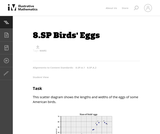Unrestricted Use
CC BY
Rating
0.0 stars

This task asks students to glean contextual information about bird eggs from a collection of measurements of said eggs organized in a scatter plot. In particular, students are asked to identify a correlation and use it to make interpolative predictions, and reason about the properties of specific eggs via the graphical presentation of the data.

Subject:
Mathematics
Statistics and Probability
Material Type:
Activity/Lab
Provider:
Illustrative Mathematics
Provider Set:
Illustrative Mathematics
Author:
Illustrative Mathematics
05/01/2012Unrestricted Use
CC BY
Rating
0.0 stars

This resource consists of a Java applet and expository text. The applet is a simulation of the birthday experiment: a sample of size n is chose at random and with replacement from the first m positive integers. The random variable of interest is the number of distinct sample values. The event of interest is that all sample values are distinct.

Subject:
Statistics and Probability
Material Type:
Activity/Lab
Interactive
Simulation
Provider:
University of Alabama in Huntsville
Provider Set:
Virtual Laboratories in Probability and Statistics
Author:
Kyle Siegrist
02/16/2011Unrestricted Use
CC BY
Rating
0.0 stars

This resource consists of a Java applet and expository text. The applet simulates the bivariate normal distribution. The means are set at 0, but the standard deviations and the correlation can be varied. Simulated points from the distribution are shown as dots in a scatterplot.

Subject:
Statistics and Probability
Material Type:
Activity/Lab
Interactive
Simulation
Provider:
University of Alabama in Huntsville
Provider Set:
Virtual Laboratories in Probability and Statistics
Author:
Kyle Siegrist
02/16/2011Unrestricted Use
CC BY
Rating
0.0 stars

This resource consists of a Java applet and expository text. The Java applet illustrates the bivariate uniform distribution on three types of regions: a square, a circle, and a triangle. Simulated points from the distribution are shown as dots in a scatterplot.

Subject:
Statistics and Probability
Material Type:
Activity/Lab
Interactive
Simulation
Provider:
University of Alabama in Huntsville
Provider Set:
Virtual Laboratories in Probability and Statistics
Author:
Kyle Siegrist